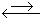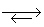Name:    Practice Test 2B

Multiple Choice

1.

What is the pH of a 0.050 M LiOH solution?
 A) 11 B) 1.3 C) 12.7 D) 3

2.

If the pH of a solution has increased from 2.0 to 4.0, the [H3O+]
 A) decreases by a factor of 10 B) increases by a factor of 100 C) increases by a factor of 2 D) decreases by a factor of 100

3.

Which of the following is NOT an acid-base conjugate pair?
 A) HCl and Cl– B) H2O and OH– C) SO42– and SO32– D) HCO3– and CO22–

4.

Which of the follwing is amphoteric in an aqueous solution?
 A) HI B) HSO3– C) H2S D) PO43–

5.

Based on the reactions below, determine the relative acid strengths (strongest to weakest).

HCl (aq) + H2O (l)H3O+ (aq) + Cl (aq)

HCN (aq) + H2O (l)H3O+ (aq) + CN (aq)
 A) H3O+ > HCl > HCN B) HCl > HCN > H3O+ C) HCN > H3O+ > HCl D) HCl > H3O+ > HCN

6.

Which of the following statements is correct given the equilibrium shown below:

H3PO4 (aq) +  NO2  (aq)H2PO4 (aq)  + HNO2 (aq)
 A) H3PO4 is a stronger acid than HNO2 B) NO2– is a weaker base than H2PO4– C) H3PO4 is a weaker acid than HNO2 D) H2PO4– anion is a stronger acid than HNO2

7.

Which of the following pairs has the weaker acid listed first?
 A) HI and HCl B) CH3COOH and CH2BrCOOH C) HClO3 and HClO2 D) HNO3 and HNO2

8.

What is the [OH]for a solution that has a pH of 4.29?
 A) 1.4 x 10–2 M B) 7.3 x 10–13 M C) 5.1 x 10–5 M D) 1.9 x 10–10 M

9.

Calculate the [H+] in a 1.0 M solution of HCN (Ka = 4.0 x 10–10).
 A) 2.0 x 10–10 B) 4.0 x 10–10 C) 2.0 x 10–5 D) 2.0 x 10–8

10.

Which acid below has the strongest conjugate base?
 A) HF B) HBr C) HClO4 D) HI

11.

Which of the following solutions contains the strongest acid?
 A) 0.1 M HA, pH = 6.85 B) 0.1 M HD, pH = 7.22 C) 0.1 M HE, pH = 4.34 D) 0.1 M HJ, pH = 8.34

12.

Which of the following acids is the weakest?
 A) HA, Ka = 6.8 x 10–8 B) HM, Ka = 3.4 x 10–6 C) HJ, Ka = 2.1 x 10–5 D) HZ, Ka = 3.0 x 10–10

13.

A solution is prepared by adding 0.10 mol of Fe(NO3)3 to 1 L of water.  Which of the following statements is true?
 A) The solution is basic B) The solution is acidic C) The solution is neutral D) No meaningful predictions can be made without knowing Ka and Kb values